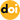### Mathematical model and vector control of a six-phase linear induction motor with the dynamic end effect

Vol. 20, No. 3, pp. 698-709, May  202010.1007/s43236-020-00059-x

###### Abstract

This study investigates a short primary double-side six-phase linear induction motor (LIM) operating in non-periodic transient conditions. The main purpose is to solve the problems arising from the dynamic end effect. The equivalent circuit method is used for intensively studying the transient time-varying law of eddy current and excitation inductance, and the finite element method is adopted for verification. The calculation of eddy current loss leads to an expression of secondary equivalent resistance. Then, a mathematical model is established for the six-phase LIM involving the dynamic end effect. Furthermore, the matrix reconstruction method is adopted for the coupling of windings. Based on the time-varying characteristics of the model, an improved vector control method that can update the velocity-related correction coefficients through on-line calculation is proposed. During the transient process, the slip frequency and the torque current are modified in real-time to achieve the maximum output thrust of the motor. This approach can compensate for the thrust drop caused by the dynamic end effect, thereby improving the control precision. Simulations and experiments show that the related theory and the proposed control method are feasible and effective.

###### Statistics
Show / Hide Statistics

Cumulative Counts from September 30th, 2019
Multiple requests among the same browser session are counted as one view. If you mouse over a chart, the values of data points will be shown.

Cite this article

[IEEE Style]

Y. Han, Z. Nie, J. Xu, J. Zhu and J. Sun, "Mathematical model and vector control of a six-phase linear induction motor with the dynamic end effect," Journal of Power Electronics, vol. 20, no. 3, pp. 698-709, 2020. DOI: 10.1007/s43236-020-00059-x.

[ACM Style]

Yi Han, Ziling Nie, Jin Xu, Junjie Zhu, and Jun Sun. 2020. Mathematical model and vector control of a six-phase linear induction motor with the dynamic end effect. Journal of Power Electronics, 20, 3, (2020), 698-709. DOI: 10.1007/s43236-020-00059-x.